# Ghost Busters – Imposter Edition 👀

### Inspiration

When I was in 10th grade, I really got into ghost-busting shows. The thrill of seeing people go to renowned places of horror was amazing. Especially when none of it had anything to do with me, as I sit comfortably in my couch.

One of the devices that caught my attention appears in the show Buzzfeed Unsolved.  When the hosts Ryan Bergara and Shane goes into places that are suspected to have entities, they open a sound-making device called the “spirit box”. The spirit box allegedly goes through multiple radio channels at a rapid pace, producing a sort of white noise. In this white noise, it is believed that ghost and entities would be able to make some sort of sound to communicate with the living

### Specifics

For my project, I wanted to make a device that mimics what the spirit box does, adding some additional features. The system is composed of an Arduino and my laptop (processing). On the Arduino, there are two systems.

The detection system includes a photoresistor and an ultrasonic distance sensor. When the user walks into a haunted place, the photoresistor will notice the light getting darker, giving it an indication that there is a possibility for ghost activity. Then, the distance sensor will detect whether the user is close to a corner, wall, or object. These are all areas that are likely to have paranormal activity. There is also a piezo disk on the Arduino. While detecting, the piezo disk will inconsistently beep. There’s no particular use for it, just to add tension and mimic the original spirit-box.

Once an activity is detected, the box sends the signal to the controlling centre (laptop/processing). The box is able to detect what type of ghost it is as well. A good one, a neutral one, or an evil one.

On processing, there is a “game” that is used to “release” the ghost from its half-dead state. The game difficulty varies based on the type of ghost. The game also utilizes the second system on the Arduino, the “control system”. This is composed of a knob (potentiometer) and a button.

### The Game

The Game layouts look like this

Good ghost: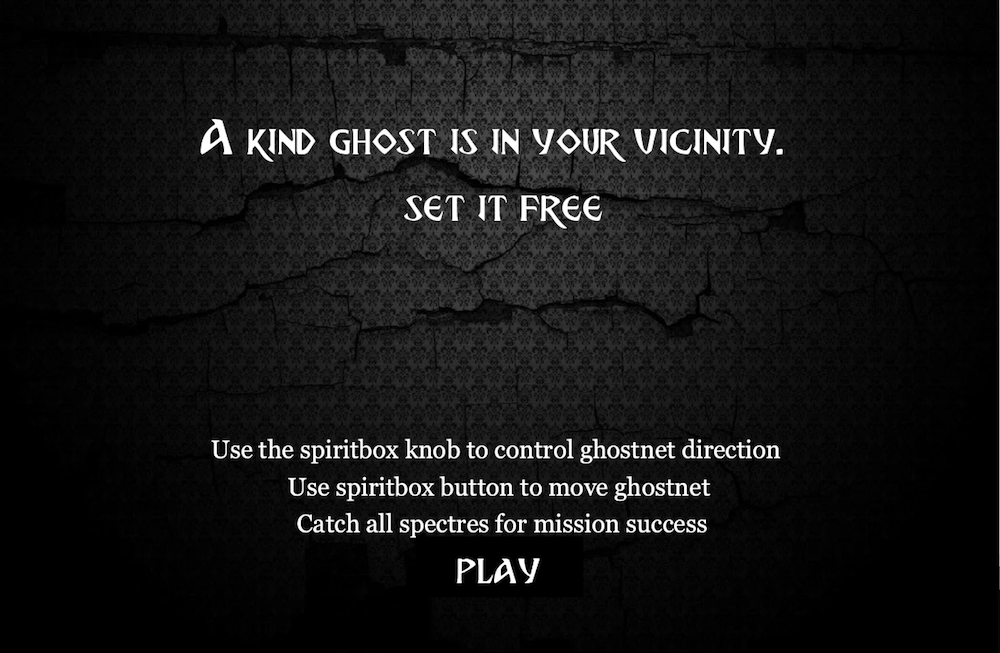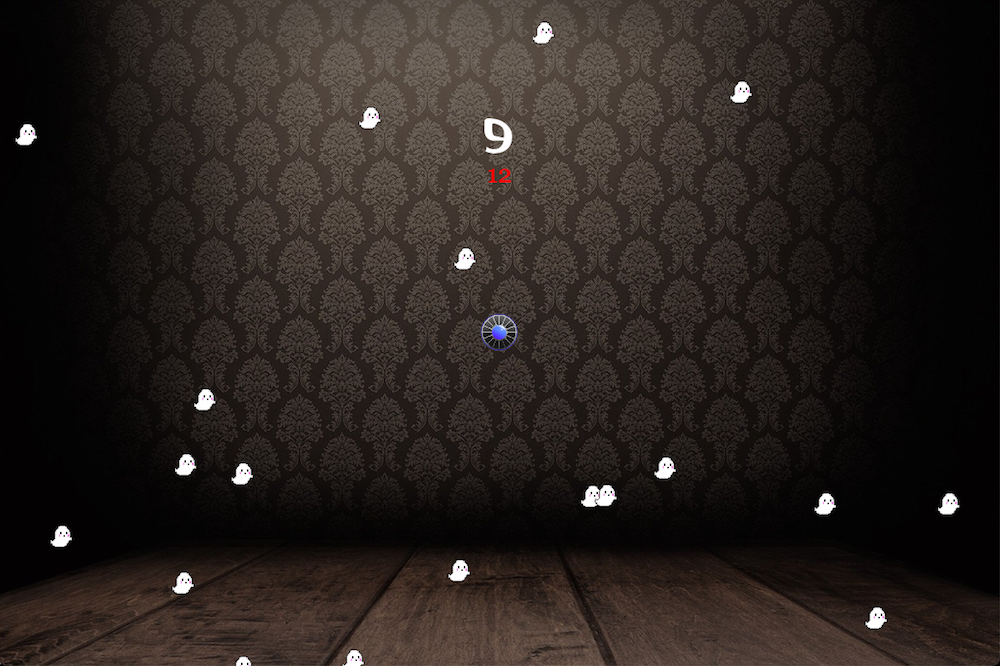Neutral ghost: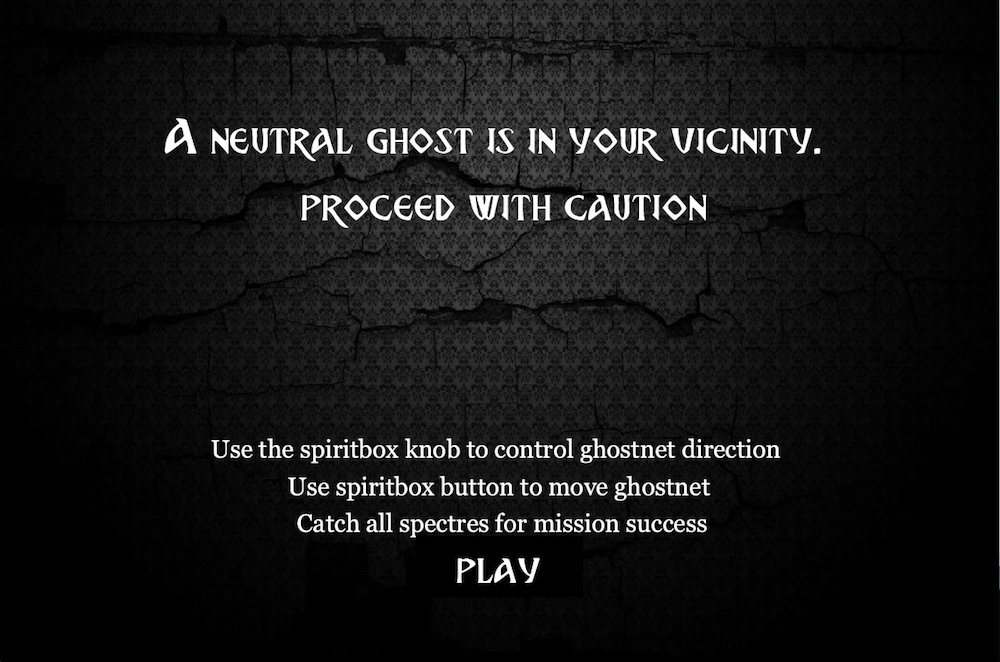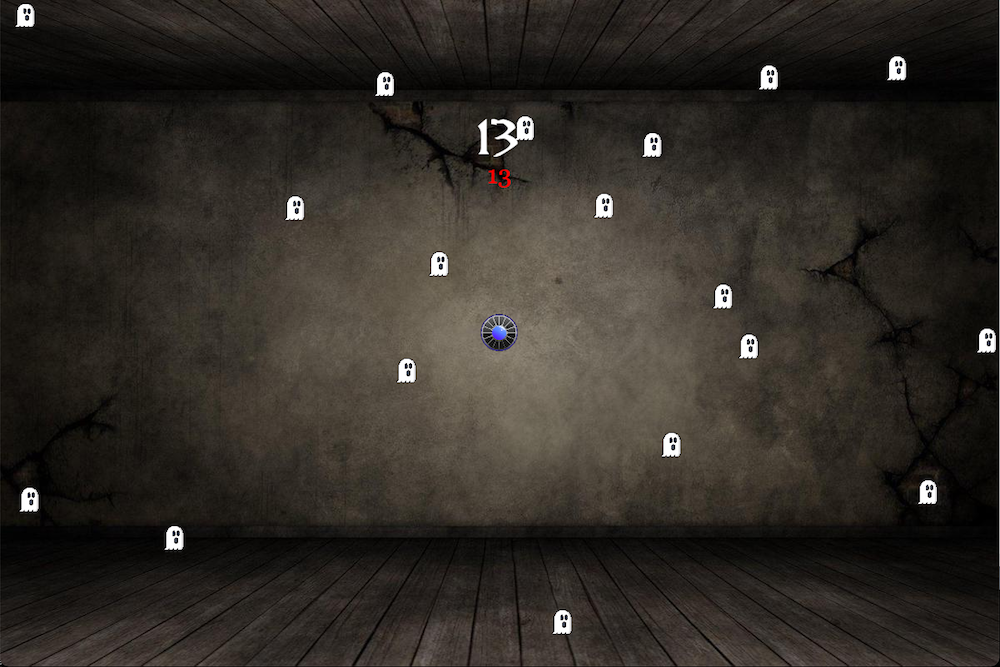Evil ghost: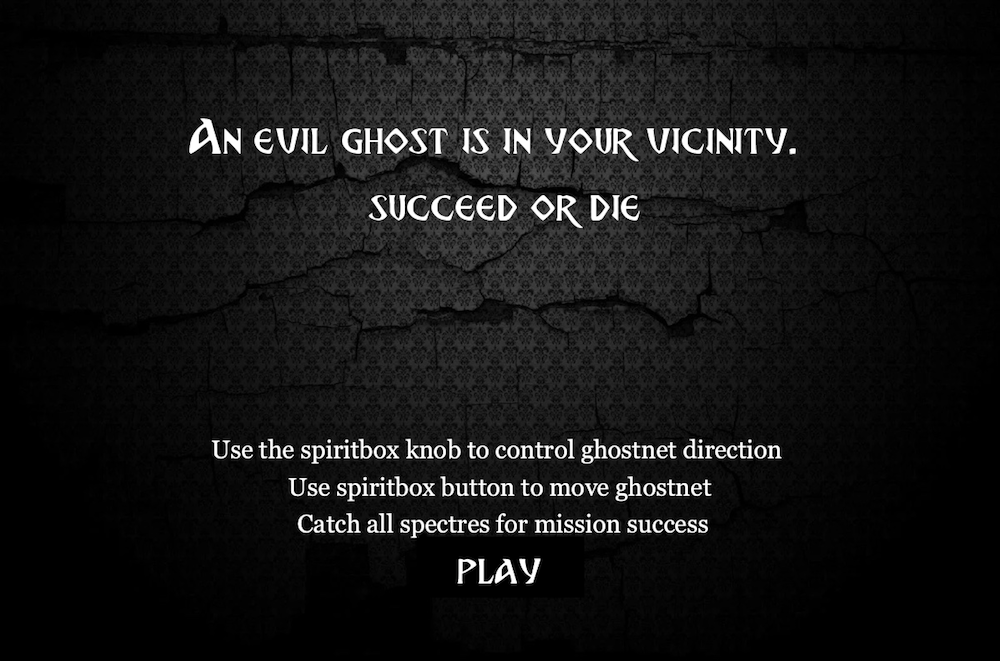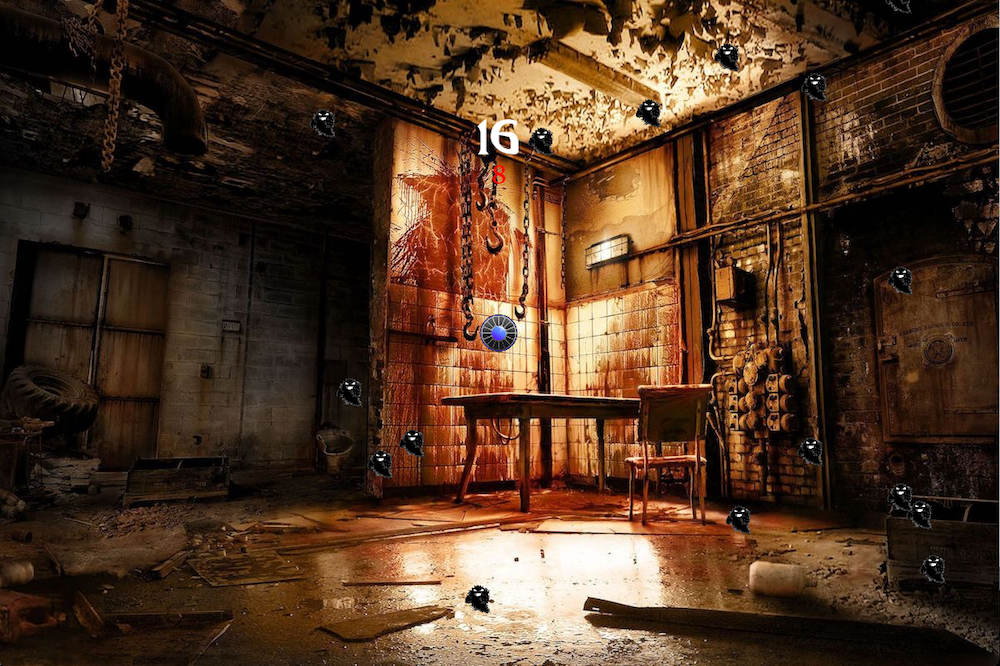The disk in the middle is a “ghostnet”. To control the ghost net, the players use the “control system” on the Arduino. The knob controls direction, and the button controls move or stop. There are 20 spectres every time a ghost is caught, and the number of spectres required to be caught depends on the “evilness” of the ghost. A certain amount of time is given to the players to finish this task, and a red countdown is displayed on the screen. A count for spectres left to catch is also displayed on the screen in white.Fail Screen: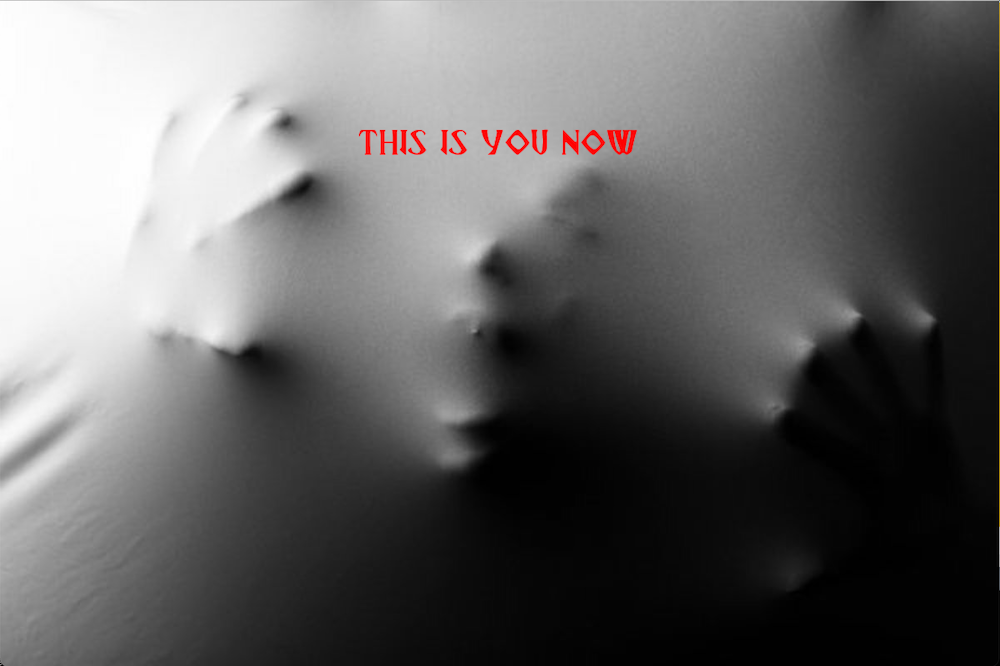Arduino Layout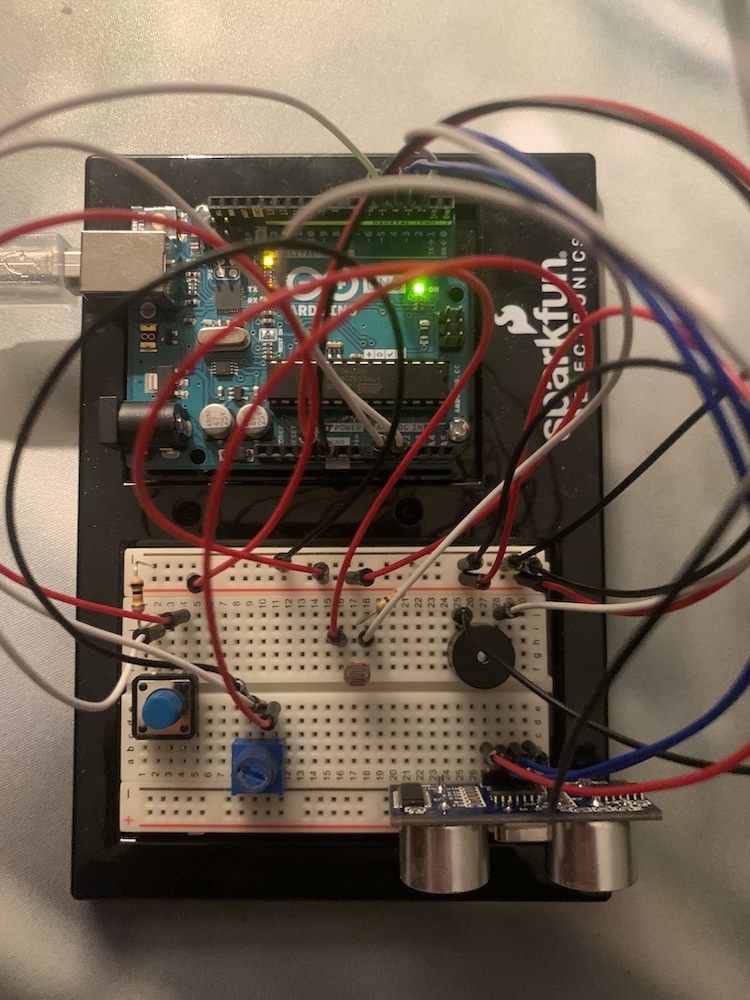### Pitfalls and Future Improvements

One of the main troubles that I ran into was the control system for the game. The knob and the buttons were a bit hard to use for the game. Although I originally intended for the controls in the game to be harder, I had to lower the difficulty holistically to account for the difficult control system.

Another difficulty and potential improvement was the mobility of the system. To use the system, the user has to bring a laptop, while taking care of the wire between the Arduino and the computer. For future purposes, the connection between the platforms could be made wireless.

### Full Code

#### Arduino

```#include "pitches.h"

int piezo = 2;
bool piezoState = false;
int trig = 3;
int echo = 4;
int button = 5;
int buttonState = 0;
int prevButton = 0;
long timer;
int timerlength = 100;
int degree;

//ghost types
bool good = false;
bool neutral = false;
bool evil = false;
int ghostType = 0;

int ghost = 0;

void setup() {
Serial.begin (9600);
Serial.write(0);
timer = millis() + timerlength;
pinMode(trig, OUTPUT);
pinMode(echo, INPUT);
pinMode(button, INPUT);

}

void loop() {

Piezo();
if (good == false && neutral == false && evil == false){
Evaluate();
}
else{
noTone(piezo);
knobValue /= 4;
degree = map(knobValue, 0, 255, 0, 12);
Serial.print(degree);
Serial.print(',');
Serial.println(buttonState);
}
}

void Evaluate() {
// For Distance
int time;
int distance;

digitalWrite(trig, HIGH);
delay(0.01);
digitalWrite(trig, LOW);

time = pulseIn(echo, HIGH);

//  speed of sound = 343 m/s = 0.0343 cm/µs
//  distance = time * speed
distance = time * 0.0343 / 2;
//  Serial.print(distance);
//  Serial.println(" cm");

// For brightness

//overall evaluation
if (millis()%10 == 1 && (Serial.available()>0)){
//    Serial.println("here");
if (brightness <= 40 && brightness > 30 && distance <= 30 && distance > 20){
ghost = random(1, 101);
if (ghost < 2){
good = true;
Serial.write(ghost);
}
}
if (brightness <= 20 && brightness > 10 && distance <= 20 && distance >10){
ghost = random(1,101);
if (ghost = 1){
good = true;
Serial.write(ghost);
}
if (ghost = 2){
neutral = true;
Serial.write(ghost);
}
}
if (brightness <= 10 && distance <= 10){
ghost = random(1,101);
if (ghost == 1){
good = true;
Serial.write(ghost);
}
if (ghost == 2){
neutral = true;
Serial.write(ghost);
}
if (ghost == 3){
evil = true;
Serial.write(ghost);
}
}
}
}

void Piezo() {
if (good == false && neutral == false && evil == false){
if (millis() > timer){
tone(piezo, C5);
timer = millis() + timerlength;
}
else if (millis() == timer){
noTone(piezo);
}

}
}

```

#### Processing

Main code

```import processing.serial.*;
Serial myPort;

import processing.sound.*;
SoundFile die;
SoundFile music2;

PFont font;

//images
PImage bg;
PImage bg1;
PImage bg2;
PImage bg3;
PImage good;
PImage neutral;
PImage evil;
PImage net;

//values:
int ghost = 0;
boolean go = false;
int ndegree = 0;
int fire = 0;
boolean win = false;

//objects:
Net n1;
Spectre[] spectre;                        //initializing all the spectre
int count = 20;
int livecount;

void setup(){
size(1200,800);

printArray(Serial.list());
String portname=Serial.list();
println(portname);
myPort = new Serial(this,portname,9600);

die = new SoundFile(this, "die.mp3");
music2 = new SoundFile(this, "music2.mp3");

//initializing objects
n1 = new Net(width/2, height/2);
spectre = new Spectre[count];
int index = 0;
for (int x = 0; x < count; x++) {
spectre[index++] = new Spectre(random(0, width), random(0, height), 40, 40);
}

for (int i =0; i<count;i++){                     //spectre speed initializing
spectre[i].xSpeed = random(-2,2);
spectre[i].ySpeed = random(-2,2);
}
music2.loop();
}

void draw(){
background(0);
//detection phase
if (ghost == 0){
font = createFont("BreatheFire-65pg.ttf", 60);
textFont(font);
textAlign(CENTER);
text("detecting . . .", width/2, 350);
}

//detected
if (ghost != 0){

}
if (go == true){
game();
checkwin();
}

}

void game(){
if (ghost == 1){
background(bg1);
}
if (ghost == 2){
background(bg2);
}
if (ghost == 3){
background(bg3);
}
n1.display();
n1.move();
n1.checkEdges();
for (Spectre spectre : spectre) {
spectre.display();
spectre.collision();
spectre.update();
spectre.checkEdges();
}
font = createFont("BreatheFire-65pg.ttf", 60);
textFont(font);
textAlign(CENTER);
text(livecount, width/2, 185);
}

if (go == false){
background(bg);
fill(255);
font = createFont("BreatheFire-65pg.ttf", 60);
textFont(font);
textAlign(CENTER);
if (ghost == 1){
text("A kind ghost is in your vicinity. \n set it free", width/2, 185);
count = 10;
}
if (ghost == 2){
text("A neutral ghost is in your vicinity. \n proceed with caution", width/2, 185);
count = 15;
}
if (ghost == 3){
text("An evil ghost is in your vicinity. \n succeed or die", width/2, 185);
count = 20;
}
livecount = count;
font = createFont("Georgia", 40);
textFont(font, 30);
text("Use the spiritbox knob to control ghostnet direction \n Use spiritbox button to move ghostnet \n Catch all spectres for mission success", width /2, 550);
stroke(0);
fill(0);
rectMode(CENTER);
rect(width/2, 680, 200, 72);
fill(255);
textFont(font, 60);
font = createFont("BreatheFire-65pg.ttf", 42);
textFont(font);
textAlign(CENTER);
text("PLAY", width/2, 700);
if (mouseX>=(width/2 - 100)  & mouseX<=(width/2 + 100) & mouseY>=650 & mouseY<=720){
stroke(255);
noFill();
rect(width/2, 680, 200, 72);
if (mousePressed){
go = true;
music.stop();
}
}
}
}

void checkwin(){
if (livecount < 1){
win = true;
background(bg);
fill(255);
font = createFont("BreatheFire-65pg.ttf", 60);
textFont(font);
textAlign(CENTER);
text("mission success \n report back to Jacob", width/2, 185);
myPort.write(int(win));
}
}

void serialEvent(Serial myPort){
if (ghost == 0){
}
else if (ghost != 0){
s=trim(s);
if (s!=null){
int values[]=int(split(s,','));
if (values.length==2){
//println(values);
ndegree = values;
fire = values;
}
}
}
myPort.write(0);
}```

Ghost Net Class

```class Net {

float x;
float y;
float diameter;
int score;
float xSpeed;
float ySpeed;

Net(float tempX, float tempY) {
x = tempX;
y = tempY;
diameter = 45;
score = 0;
}

void display() {
imageMode(CENTER);
image(net,x,y,diameter,diameter);
}

void move() {
xSpeed = -(cos(ndegree) * 3);
ySpeed = - (sin(ndegree) * 3);
if (fire == 1){
x += xSpeed;
y += ySpeed;
}
}

void checkEdges() {
if (y>height) {
ySpeed = -ySpeed;
}
if (y<0) {
ySpeed = -ySpeed;
}
if (x>width) {
xSpeed = -xSpeed;
}
if (x<0) {
xSpeed = -xSpeed;
}
}
}```

Spectre Class

```class Spectre {
float x;
float y;
float w;
float h;;
float xSpeed, ySpeed;
boolean caught = false;

Spectre(float tempX, float tempY, float tempW, float tempH) {
x = tempX;
y = tempY;
w = tempW;
h = tempH;
xSpeed = ySpeed = 0;
}

void display() {
if (caught == false){
if (ghost == 1){
image(good, x, y, w, h);
}
if (ghost == 2){
image(neutral, x, y, w, h);
}
if (ghost == 3){
image(evil, x, y, w, h);
}
}
}

//collision function for when players catch the right and wrong spectre
void collision() {
if ((n1.x - n1.diameter < x && x < n1.x + n1.diameter) & (n1.y - n1.diameter < y && y < n1.y + n1.diameter)){

x = -50;
y = -50;
caught = true;
livecount --;
die.play();

}
}

void update(){
x += xSpeed;
y += ySpeed;
}

void checkEdges() {
if (y>height) {
ySpeed = -ySpeed;
}
if (y<0) {
ySpeed = -ySpeed;
}
if (x>width) {
xSpeed = -xSpeed;
}
if (x<0) {
xSpeed = -xSpeed;
}
}
}```

## Finals Update – Nathan

### Arduino Board

Currently, I have wired up the Arduino with everything I want to include in my final. It includes:

• An ultrasonic distance sensor
• A piezo
• A photoresistor
• A button
• A knob (potentiometer)

Here is a picture of the layout: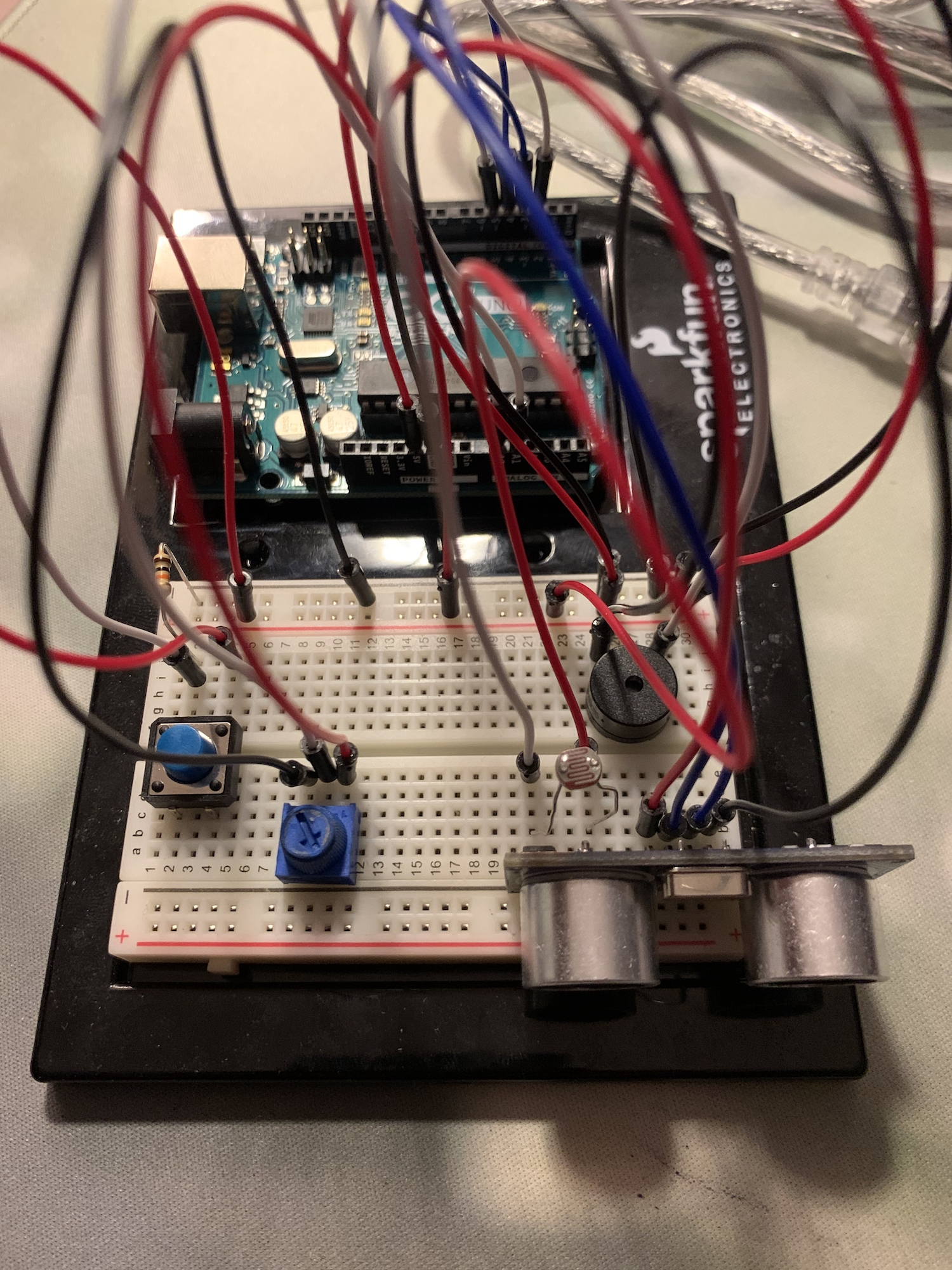### Code

For code, I currently have coded in the conditions for when the piezo is making sounds, as well as how its sound is being made. I also have the distance sensor and photoresistor coded in as functions, but I have not fully integrated them with the system in general.

I haven’t gotten to the processing side of the code yet, but I am planning to make several boolean values on the Arduino side to represent the signals being sent to processing. For example, if there is a friendly ghost, I will simply send the boolean to processing. I think this approach simplifies communication between the platforms. In my opinion, the more complicated alternative would be to send the raw values of the sensors and buttons on the Arduino to processing.

Here is the code I have now:

```#include "pitches.h"

int piezo = 2;
bool piezoState = false;
int trig = 3;
int echo = 4;
int button = 5;
int buttonState = 0;
int prevButton = 0;
//photoresistor A0;
//knob = A1;
long timer;
int timerlength = 200;

void setup() {
Serial.begin (9600);
timer = millis() + timerlength;
pinMode(trig, OUTPUT);
pinMode(echo, INPUT);
pinMode(button, INPUT);
}

void loop() {

////  Serial.println(knobValue);

Piezo();
}

void Piezo() {
if (piezoState == false){
if (millis() > timer){
tone(piezo, C5);
timer = millis() + timerlength;
}
else if (millis() == timer){
noTone(piezo);
}
}

if (buttonState == HIGH && prevButton == LOW){
piezoState = true;
}

if (piezoState == true){
noTone(piezo);
}

prevButton = buttonState;
}

void Brightness(){
int brightness;

return brightness;
}

void Distance() {
int time;
int distance;

digitalWrite(trig, HIGH);
delay(0.01);
digitalWrite(trig, LOW);

time = pulseIn(echo, HIGH);

//  speed of sound = 343 m/s = 0.0343 cm/µs
//  distance = time * speed
distance = time * 0.0343 / 2;
Serial.print(distance);
Serial.println(" cm");

return distance;
}```

## Midterm Refined

### Inspiration

For this week’s project, I wanted to use Arduino controls to refine what I did in my midterm. To refresh your memory, my midterm was a two-player game where the players ran around the map competing for candies. The game was ok, but the main bug was that I couldn’t have the two players moving at one time. This was due to the limitations of “Keypressed function”, as it wasn’t able to differentiate which player was pressing the keys.

By introducing Arduino into the game, I could simply create another “console” for player 2, so the player controls won’t be mixed up in the game.

### Limitations

There were two limitations to using Arduino as a “console.” One is more obvious, which is the fact that the kit buttons aren’t that responsive, and aren’t really stationary. While I was playing the game with it, the buttons would slip off a lot of the times.

The second limitation is the problem of the breadboard. There are four buttons in the kit, so just enough for the four directions. However, the breadboard didn’t have the horizontal space to put an “up” and “down” button next to each other. As a compromise, I had to put the four buttons in a row, with the middle right being “up” and middle left “down”. Here is the final layout: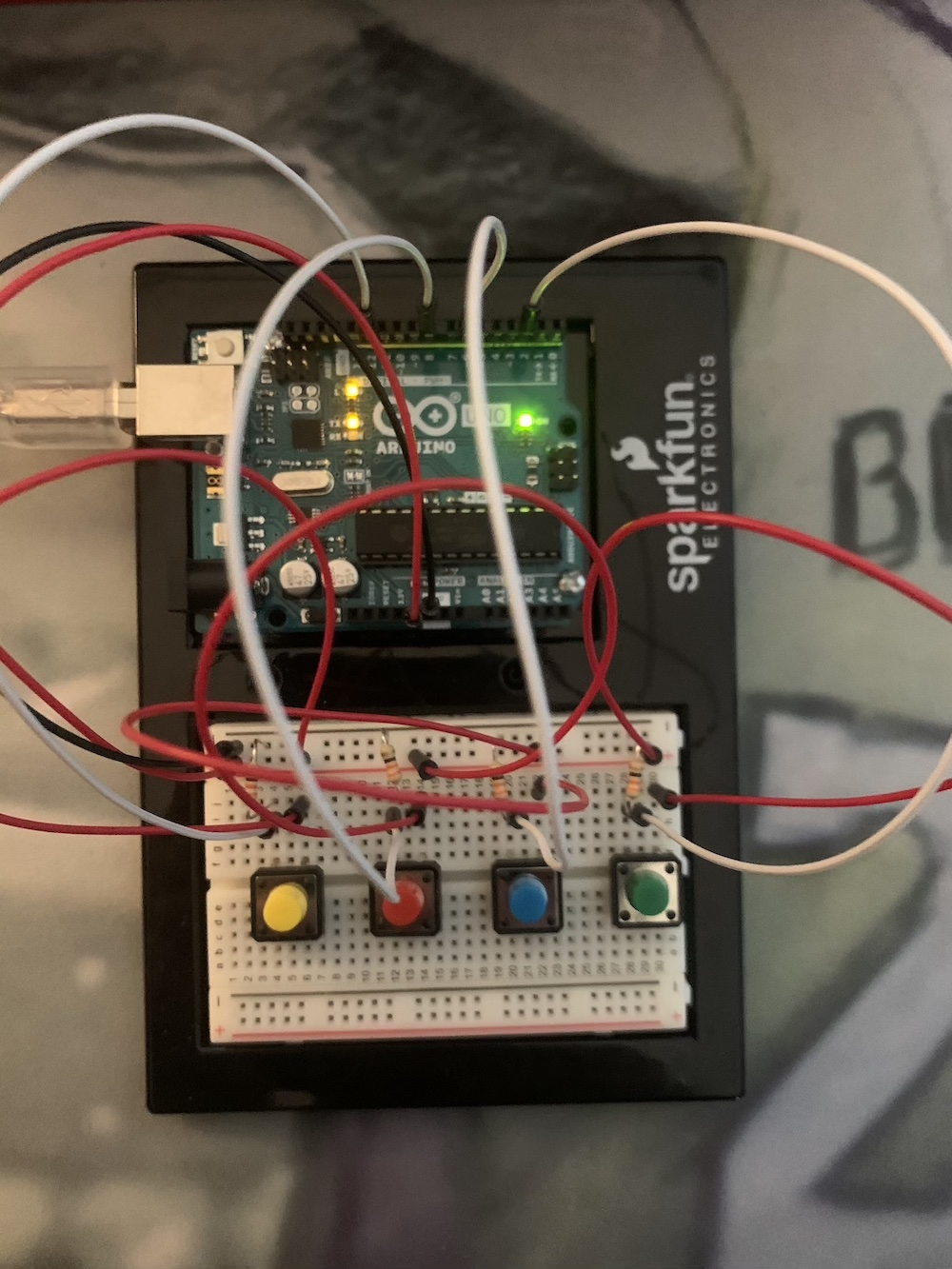### Challenges

The main challenge I ran into for this project was trying to integrate Arduino into my midterm project code. My midterm code was rather chaotic with a lot of functions, so it took a lot of time trying to piece together where the Arduino parts of the code would fit into the logic.

### Full code

Arduino:

```int button1 = 2;
int button2 = 12;
int button3 = 6;
int button4 = 8;

void setup() {
// put your setup code here, to run once:
pinMode(button1, INPUT);
pinMode(button2, INPUT);
pinMode(button3, INPUT);
pinMode(button4, INPUT);
Serial.begin(9600);
}

void loop() {
Serial.print(buttonState1);
Serial.print(',');
Serial.print(buttonState2);
Serial.print(',');
Serial.print(buttonState3);
Serial.print(',');
Serial.println(buttonState4);
}```

Main Code:

```PFont font;

import processing.serial.*;
Serial myPort;
boolean left = false;
boolean right = false;
boolean up = false;
boolean down = false;

import processing.sound.*;            //all the sounds in this game
SoundFile eat;
SoundFile die;
SoundFile music;

Ball b1;                              //initializing the two players
Ball b2;
PImage ninja, warrior, bg;
PImage[][] ninjawalk;
PImage[][] warriorwalk;
int ndirection = 0;
int wdirection = 0;
int nstep = 0;
int wstep = 0;
int speed = 5;

Candy[] candy;                        //initializing all the candy
int count;
color chosen;
int livecount = 0;                    //how many edible candy there are

boolean go = false;                   //start game value

void setup() {
size(1200, 800);

printArray(Serial.list());
String portname=Serial.list();
println(portname);
myPort = new Serial(this,portname,9600);
myPort.clear();
myPort.bufferUntil('\n');

//sounds
eat = new SoundFile(this, "eat.mp3");
die = new SoundFile(this, "die.mp3");
music = new SoundFile(this, "music.mp3");
music.loop();

//slicing the two sprite sheets for player 1 and 2
ninjawalk = new PImage;
int w = ninja.width/3;
int h = ninja.height/4;
for(int iy = 0; iy < 4; iy++){
for(int ix = 0; ix<3; ix++){
ninjawalk[iy][ix] = ninja.get(ix*w, iy *h, w, h);
}
}
warriorwalk = new PImage;
int ww = warrior.width/3;
int wh = warrior.height/4;
for(int wy = 0; wy < 4; wy++){
for(int wx = 0; wx<3; wx++){
warriorwalk[wy][wx] = warrior.get(wx*ww, wy *wh, ww, wh);
}
}
//use this function to restart the game
reset();
}

void draw() {
if (go == true){
begin();
}
}

void serialEvent(Serial myPort){
s=trim(s);
if (s!=null){
int values[]=int(split(s,','));
if (values.length==4){
println(values);
if (values == 1){
right = true;
}
else if (values == 0){
right = false;
}
if (values == 1){
left = true;
}
else if (values == 0){
left = false;
}
if (values == 1){
up = true;
}
else if (values == 0){
up = false;
}
if (values == 1){
down = true;
}
else if (values == 0){
down = false;
}
}
}
}

//this function restarts the game from the gaming interface directly
void reset(){
float unit = 200;
b1 = new Ball(width/4, height - 20, 1);          //player 1 character
b2 = new Ball(width*3/4, height - 20, 2);        //player 2 character

int wideCount = width / int(unit);               //calling the candies, initializing different candy colors
int highCount = (height*3/4) / int(unit);
count = wideCount * highCount;
candy = new Candy[count];
int index = 0;
color[] colors = new color;
colors = #FF0011;
colors = #BA00FF;
colors = #2500FF;
colors = #00FFE8;
colors = #00FF01;
colors = #FFAF00;
chosen = colors[int(random(0,5))];
for (int y = 0; y < highCount; y++) {
for (int x = 0; x < wideCount; x++) {
candy[index++] = new Candy(x * unit + unit/2, y * unit + unit/2, 20, 20, colors[int(random(0,5))]);
}
}

for (Candy candy : candy) {                      //counting how many edible candies there are to know the win condition
if (chosen == candy.c){
livecount ++;
}
}

for (int i =0; i<count;i++){                     //candy speed initializing
candy[i].xSpeed = random(-2,2);
candy[i].ySpeed = random(-2,2);
}
}

background(bg);
fill(255);
font = createFont("BreatheFire-65pg.ttf", 60);
textFont(font);
textAlign(CENTER);
text("CANDY RUSH \n even heros like candy", width/2, 185);
font = createFont("Georgia", 40);
textFont(font, 30);
text("This is a two player game. Ninja and Warrior wants to take a break \n on their quest, so they are going to catch candy. Some candies are \n poisonous, and only the candy indicator can tell you which candies are edible. \n The first player to catch all the edible candy wins. \n NINJA: WASD, WARRIOR: direction keys", width /2, 370);
stroke(0);
fill(0);
rectMode(CENTER);
rect(width/2, 680, 200, 72);
fill(255);
textFont(font, 60);
font = createFont("BreatheFire-65pg.ttf", 42);
textFont(font);
textAlign(CENTER);
text("PLAY", width/2, 700);
if (mouseX>=(width/2 - 100)  & mouseX<=(width/2 + 100) & mouseY>=650 & mouseY<=720){
stroke(255);
noFill();
rect(width/2, 680, 200, 72);
if (mousePressed){
go = true;
}
}
}

//this is the game starting function. the actual game is ran here
void begin(){
background(bg);
textSize(24);
text(b1.score, width/4, height - 50);
text(b2.score, width*3/4, height - 50);

//calling candy functions
for (Candy candy : candy) {
candy.display();
candy.collision();
candy.update();
candy.checkEdges();
}

//this is the candy indicator
stroke(0);
fill(chosen);
ellipse(width/2, height - b1.diameter, b1.diameter, b1.diameter);

//calling character functions
b1.display();
b1.move();
b2.display();
b2.move();
checkwin();
}

void checkwin(){
if (livecount == 0){                      //win condition
for (Candy candy : candy) {
candy.xSpeed = 0;
candy.ySpeed = 0;
}
rectMode(CENTER);
if (b1.score > b2.score){
fill(0);
rect(width/2, height/2, width, height);
fill(255, 255, 255);
textSize(32);
text("Ninja Wins! \n (Press r to restart)", width/2, height/2);
}
else if (b2.score > b1.score){
fill(0);
rect(width/2, height/2, width, height);
fill(255, 255, 255);
textSize(32);
text("Warrior Wins! \n (Press r to restart)", width/2, height/2);
}
else if (b2.score == b1.score){
fill(0);
rect(width/2, height/2, width, height);
fill(255, 255, 255);
textSize(32);
text("Tie! \n (Press r to restart)", width/2, height/2);
}

//restart button
if (keyPressed) {
if (key == 'r' || key == 'R') {
reset();
}
}
}
}```

Player Class:

```class Ball {

float x;
float y;
float diameter;
int score;
int player;
int w = ninja.width/3;
int h = ninja.height/4;
int ww = warrior.width/3;
int wh = warrior.height/4;

Ball(float tempX, float tempY, int tempPlayer) {
x = tempX;
y = tempY;
diameter = 45;
score = 0;
player = tempPlayer;
}

void display() {
imageMode(CENTER);                                    //displaying player 1
if (player == 1) {
image(ninjawalk[ndirection][nstep], x, y, w, h);
}
if (player == 2) {                                     //displaying player 2
image(warriorwalk[wdirection][wstep], x, y, ww, wh);
}
}

void move() {
if (player == 1) {
if (keyPressed) {
if (key == 'w' || key == 'W') {
y = y - 5;
ndirection = 0;
}
if (key == 'a' || key == 'A') {
x = x - 5;
ndirection = 3;
}
if (key == 's' || key == 'S') {
y = y + 5;
ndirection = 2;
}
if (key == 'd' || key == 'D') {
x = x + 5;
ndirection = 1;
}
if (frameCount%speed == 0) {
nstep = (nstep + 1) %3;
}
}
}

if (player == 2) {
if (up == true) {
y = y - 5;
wdirection = 0;
}
if (left == true) {
x = x - 5;
wdirection = 3;
}
if (down == true) {
y = y + 5;
wdirection = 2;
}
if (right == true) {
x = x + 5;
wdirection = 1;
}
if (frameCount%speed == 0) {
wstep = (wstep + 1) %3;
}
}
}
}```

Candy Class:

```class Candy {
float x;
float y;
float w;
float h;
float xSpeed, ySpeed;
boolean caught = false;
color c;

Candy(float tempX, float tempY, float tempW, float tempH, color tempC) {
x = tempX;
y = tempY;
w = tempW;
h = tempH;
c = tempC;
xSpeed = ySpeed = 0;
}

void display() {

ellipseMode(CENTER);
if (caught == false){
fill(c);
ellipse(x, y, w, h);
}
}

//collision function for when players catch the right and wrong candy
void collision() {
if ((b1.x - b1.w *3/4 < x && x < b1.x + b1.w*3/4) & (b1.y - b1.h*3/4 < y && y < b1.y + b1.h*3/4)){
if (c == chosen){
x = -50;
y = -50;
caught = true;
b1.score ++;
livecount --;
eat.play();
}
else if (c != chosen){
b1.x = width/4;
b1.y = height - b1.diameter;
die.play();
}
}
if ((b2.x - b2.w*3/4 < x && x < b2.x + b2.w*3/4) & (b2.y - b2.h*3/4 < y && y < b2.y + b2.h*3/4)){
if (c == chosen){
x = -50;
y = -50;
caught = true;
b2.score ++;
livecount --;
eat.play();
}
else if (c != chosen){
b2.x = width*3/4;
b2.y = height - 20;
die.play();
}
}
}

void update(){
x += xSpeed;
y += ySpeed;
}

void checkEdges() {
if (y>(height*3)/4) {
ySpeed = -ySpeed;
}
if (y<0) {
ySpeed = -ySpeed;
}
if (x>width) {
xSpeed = -xSpeed;
}
if (x<0) {
xSpeed = -xSpeed;
}
}
}```

## Nathan – New Final Project Idea

### General Idea

For my final project, I plan to do a “ghost busting” device. The device would include an Arduino layout and a laptop. When there’s a “ghost” caught, the laptop would display the type of ghost caught, its nature (malicious/neutral/friendly), and instructions on how to dispose of it. The way the player would dispose of the ghost would include a certain set of actions on the arduino.

### Inspiration

For this idea, I was inspired by watching BuzzFeed unsolved 😅. The hosts Ryan and Shane use this device called a spirit box. When they turn the box on, it makes a screeching noise produced by switching channels (radio channels I think?) at a rapid pace. It’s believed that this way, the ghosts would be able to “communicate” through the white noise. Something like this: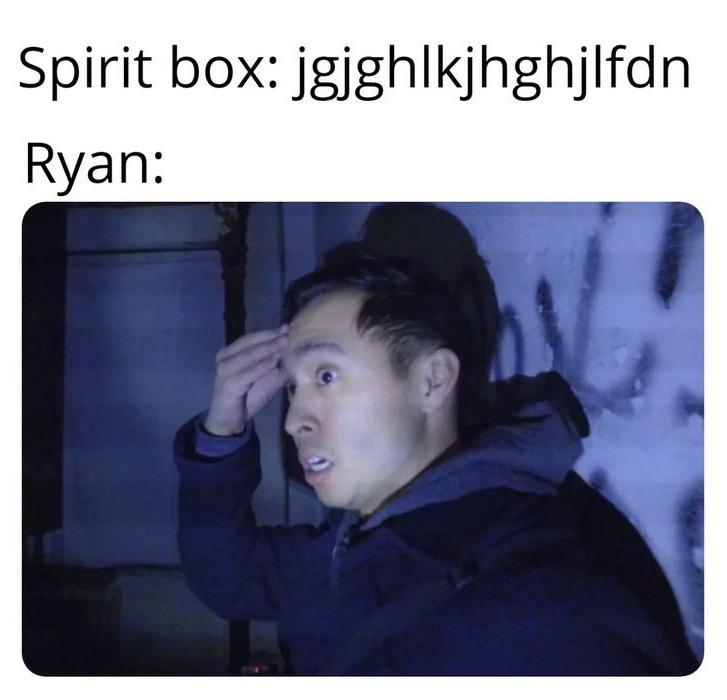Since I need to have some form of communication between the Arduino and processing for this project, I thought I might make an “upgraded” spirit box that consisted of a laptop as well.

### Specifics

Arduino components:

1. Sensing department
1. Photoresistor
1. The lower the brightness of the room, the likelier for there to be a ghost
2. Ultrasonic distance sensor
1. The closer the player is to a wall or corner, the likelier for there to be a ghost
3. Piezo disk
1. The sound changes based on the situation. No ghost is a sound, each type of ghost has a sound. (malicious has a more scary sound, friendly is more meme sound)
2. Hands-on department
1. Buttons and knob
1. For the players to interact with after encountering a ghost.

Arduino-Processing Communication:

• The photoresistor and the ultrasonic sensor will send two data values to processing, and processing will have a function that interprets the data. The chance of a ghost popping up will correlate with these data values.
• For the piezo disk, the Arduino will have four sounds coded in. (No ghost, three other types). According to the situation, processing sends a message to Arduino to play which sound.
• For the hands-on department, three sets of instructions will have been coded into the Arduino. According to the situation, Processing sends a message to Arduino for which set of actions is required.

## City of Stars ONLY player

For this week’s instrument assignment, I originally planned to make something similar to Ons and David’s project, where I use the ultrasonic proximity sensor to make a “keyboard” where I can play notes and music. However, as I saw their projects in class, I thought I might change mine up a little bit to avoid having similar projects.

First, my original idea was to have a digital input button that triggered sound whenever it was pressed. The sound played will be decided by the distance sensed by the ultrasonic proximity sensor. The distances correlated with an octave of notes.

A problem that I noticed with this approach, however, was that the music I played was usually chopped up. The interface required me to constantly remember where I have to put the “distance blocker”, and the sound of pressing the button interfered with the song every time I pressed it.

Due to these concerns, I decided that I want my project to just play ONE song, and the distance measurements won’t correlate to notes in order, but the order of the notes in the song. This way, as the “distance blocker” moves further, the song just progresses by itself, and the player could continue to hold down the button.

### Tweaks

As I re-designed my project, one obvious problem that would arise is the length of the interface. The song I want to play is City of stars. To code all of the song into arduino, while correlating with distance is problematic. I can’t reduce the space given to each note because of sensitivity issues, where it’d be too hard for the player to control the distance. However, giving each note enough distance would also make the interface too long, and the player wouldn’t be able to reach the end of it.

The solution I came up with is to integrate a knob. The entire rotation of the knob is separated into three parts. When the knob is in the first part, the interface is programmed to play part 1 of the song. Similarly, the second part of the knob programs the interface to play part 2, and the same for part 3. This way I could (sort of) dynamically change the interface when I’m done with a part of the song.

### Full code:

```#include "pitches.h"

int trig = 7;
int echo = 6;
int piezo = 12;
int button = 2;
int buttonState = 0;

//part 1
int notes = {G3, A3, AS3, D4, E4, F4, D4, E4, C4, D4, A3};
//part 2
int notes2 = {A3, AS3, D4, C4, D4, A3, A4, G4, D4, E4, C4};
//part 3
int notes3 = {C4, D4, A4, G4, F4, D4, 0, 0, 0, 0, 0};

void setup() {
Serial.begin (9600);
pinMode(trig, OUTPUT);
pinMode(echo, INPUT);
pinMode(button, INPUT);
}

void loop() {
int time;
int distance;

digitalWrite(trig, HIGH);
delay(0.01);
digitalWrite(trig, LOW);

time = pulseIn(echo, HIGH);

//speed of sound = 343 m/s = 0.0343 cm/µs
// distance = time * speed
// "taken from David thank you David"
distance = time * 0.0343 / 2;
//  Serial.print(distance);
//  Serial.println(" cm");

Serial.println(knobValue);

int note = map(distance, 2, 40, 0, 11);
int note2 = map(distance, 2, 40, 0, 11);
int note3 = map(distance, 2, 40, 0, 11);

if (buttonState == true){
if (knobValue <= 1023 && knobValue >= 682){
tone(piezo, notes[note]);
}
else if (knobValue < 682 && knobValue >= 341){
tone(piezo, notes2[note2]);
}
else if (knobValue>=0 && knobValue < 341){
tone(piezo, notes3[note3]);
}
}
else if (buttonState == false){
noTone(piezo);
}
}```

### Arduino Layout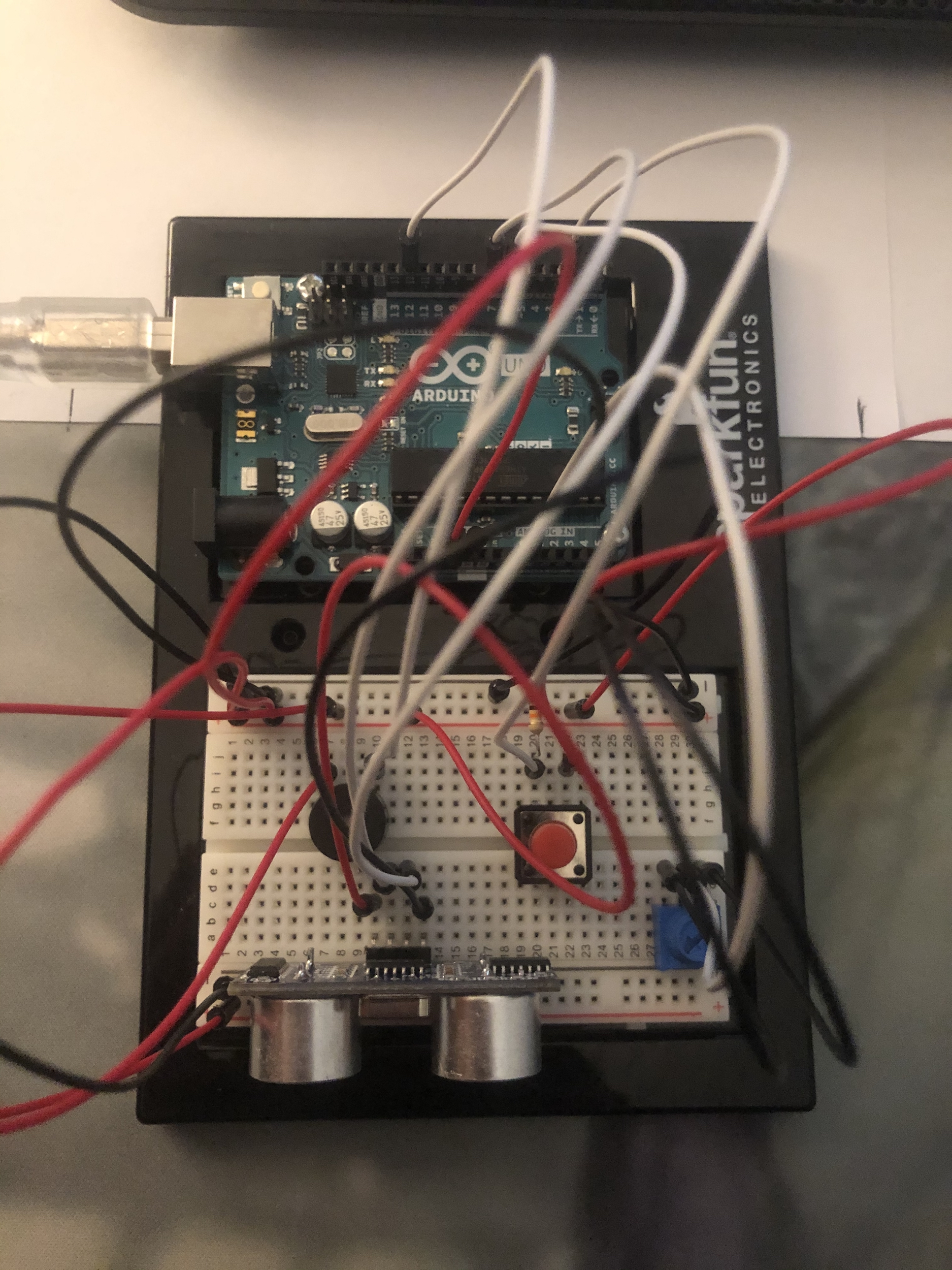## Nathan analog + digital Assignment

For this week’s assignment, I wanted to do a ghostbuster (kind of, not really) themed project. The idea is to use the potentiometer to find the position of the ghost, while the lights fade.

#### General instructions

The player is entering a house that is infested with ghosts. First, they have to click the red button on the right to activate the ghost-catching device. The ghost-catching device has five red LEDs, marking the general 5 directions in front of the player. When the lights in the house are on, all five device lights are on, as the surrounding is too bright to detect anything.

As the lights in the ghost-infested house are dimmed, the ghosts begin to reveal themselves. They are quick and barely leave marks, so the lights on the device are usually all lighting chaotically. The player then turns the knob to try and pinpoint the ghost locations.

The ghost changes its location every second. If the direction the knob is pointing to aligns with the ghost’s location, the correlating light on the device will shine, revealing that location to the player.

#### Troubles

I ran into a lot of problems for this week’s assignment. Some kind of dumb, for example, I forgot the ground the red button and was very confused about the chaotic lights that were flashing for quite some time.

I think the most useful challenge that I faced was trying to organize functions, specifically the logic of the functions and their conditions. There are many steps and phases in my project this week. When I began to code the project, I didn’t really use functions, and the loop function was a mess. So I redesigned my code and tried to compartmentalize each step. This helped a lot, but the order that the functions ran was also something that needed to be taken care of.

#### Wiring

For the wiring of this project, I had one digital button that inputs ON/OFF to the Arduino wired on the right side of the breadboard. There are five red LEDs to signify the device location lights, a potentiometer that signifies the controls for the ghost device, and a photoresistor that signifies the lights in the haunted hosue

#### Code

```int button = 2;
int led1 = 4;
int led2 = 6;
int led3 = 8;
int led4 = 10;
int led5 = 12;
bool led1state = false;
bool led2state = false;
bool led3state = false;
bool led4state = false;
bool led5state = false;
bool prevB = false;
//timer for fake ghost position
long timer;
int timerlength = 50;
//timer for real ghost position
long timer1;
int timerlength1 = 1000;
int ghostPosition;
int truePosition;

void setup() {
pinMode(button, INPUT);
pinMode(led1, OUTPUT);
pinMode(led2, OUTPUT);
pinMode(led3, OUTPUT);
pinMode(led4, OUTPUT);
pinMode(led5, OUTPUT);

Serial.begin(9600);
timer = millis() + timerlength;
timer1 = millis() + timerlength1;
}

void loop() {
deviceon();
//shifting the ghost's true position every second
if (millis() > timer1){
truePosition = random(1,6);
timer1 = millis() + timerlength1;
}
}

//function for turning the ghost catching device on
void deviceon() {
if (buttonstate == HIGH && prevB == LOW){
deviceSwitch();
}
digitalWrite(led1, led1state);
digitalWrite(led2, led2state);
digitalWrite(led3, led3state);
digitalWrite(led4, led4state);
digitalWrite(led5, led5state);
prevB = buttonstate;
lightsoff();
}

//turning the ghost device lights on or off
void deviceSwitch() {
led1state = !led1state;
led2state = !led2state;
led3state = !led3state;
led4state = !led4state;
led5state = !led5state;
}

//fake ghost position lights
void ghost() {
if (millis() > timer){
ghostPosition = random(1,6);

if (ghostPosition == 1){
digitalWrite(led1, true);
}
else if (ghostPosition != 1){
digitalWrite(led1, false);
}

if (ghostPosition == 2){
digitalWrite(led2, true);
}
else if (ghostPosition != 2){
digitalWrite(led2, false);
}

if (ghostPosition == 3){
digitalWrite(led3, true);
}
else if (ghostPosition != 3){
digitalWrite(led3, false);
}

if (ghostPosition == 4){
digitalWrite(led4, true);
}
else if (ghostPosition != 4){
digitalWrite(led4, false);
}

if (ghostPosition == 5){
digitalWrite(led5, true);
}
else if (ghostPosition != 5){
digitalWrite(led5, false);
}

timer = millis() + timerlength;
}
}

//what happens when the house lights are off
void lightsoff() {
if (light <= 250){

led1state = false;
led2state = false;
led3state = false;
led4state = false;
led5state = false;
ghost();
catching();
}
}

//function for the potentiometer, catching the true position
void catching() {
Serial.println(knobValue);
int knobPosition;

if (270 < knobValue && knobValue <= 360){
if (truePosition == 5){
led5state = !led5state;
led1state = false;
led2state = false;
led3state = false;
led4state = false;
}
}
if (360 < knobValue && knobValue <= 480){
if (truePosition == 4){
led4state = !led4state;
led1state = false;
led2state = false;
led3state = false;
led5state = false;
}
}
if (480 < knobValue && knobValue <= 650){
if (truePosition == 3){
led3state = !led3state;
led1state = false;
led2state = false;
led4state = false;
led5state = false;
}
}
if (650 < knobValue && knobValue <= 730){
if (truePosition == 2){
led2state = !led2state;
led3state = false;
led1state = false;
led4state = false;
led5state = false;
}
}
if (730 < knobValue && knobValue <= 800){
if (truePosition == 1){
led1state = !led1state;
led2state = false;
led3state = false;
led4state = false;
led5state = false;
}
}

}
```

## LED Assignment

This week, I decided to do an LED puzzle that is similar to Simon Says, where the instructions are given in the form of a melody. I didn’t want to use a song too long or else it’d be too hard, so I used the beginning of the sound of music theme song. The melody goes: 1 2 3 1 3 1 3 2 3 4 4 3 2 4.

There are four buttons on the breadboard, with four corresponding LEDs. They are Green(1), Yellow(2), Blue(3), and Red(4). When the program starts up, the lights will play (show) the melody in full. Then, it’s up to the player to recognize or memorize what the melody is. Then, the player has to press the four buttons in the order that the melody is. If they succeed in the end, all four lights will light up and flash.

In the process of doing this project, I encounter a lot of problems that were great learning experiences for Arduino. For one, when I put the condition where a button is pressed down, Arduino cycles through the execution many times, even though I just pressed it briefly. To solve this problem, I borrowed the method in class notes, where I made a “previous button” value that holds whether a button was just pressed.

There is one part of this project that I really wanted to improve on but couldn’t, which is making a database of melodies and giving them out randomly. There are a few problems that I’ll face if I want to do this. Currently, I have two arrays, one is the original melody array that is recorded when the lights are shown, and the other is the entry array of the player. I then go through a for loop the length of the original melody, and in each loop, I check whether that index is the same between the original and entry. If I wanted to do a database of melodies, I would have to find a way to make the length of the arrays dynamic and make the length of it a variable for the for-loop.

Another improvement I wanted to do was to make my code more optimized, specifically the part where the lights are showing the melody. Right now, I hardcoded the order and delay that each note should be played. What I want to do is to simply enter a dictionary of numbers, the first being the note and the second being the delay, and the code would automatically sort the dictionary into the flashing order for the lights. I ran out of time to do it this time, but I’m confident this is do-able. Getting the code to do this would also help the database idea by making it easier to code in a melody.

Here is my full code:

```//buttons and lights initialization:
int greenB = 12;
int greenL = 13;
int yellowB = 9;
int yellowL = 10;
int blueB = 6;
int blueL = 7;
int redB = 3;
int redL = 4;
int prevB = LOW;
int prevYB = LOW;
int prevBB = LOW;
int prevRB = LOW;

boolean play = true;
boolean yay = false;

int song;
int entry;
int value;
int count = 0;

void setup() {
// put your setup code here, to run once:
pinMode(greenL, OUTPUT);
pinMode(greenB, INPUT);
pinMode(yellowL, OUTPUT);
pinMode(yellowB, INPUT);
pinMode(blueL, OUTPUT);
pinMode(blueB, INPUT);
pinMode(redL, OUTPUT);
pinMode(redB, INPUT);

}

void loop() {
// put your main code here, to run repeatedly:

//instructions

if (play == true){
digitalWrite(greenL, HIGH);
delay(650);
digitalWrite(greenL, LOW);
song = 1;
digitalWrite(yellowL, HIGH);
delay(250);
digitalWrite(yellowL, LOW);
song = 2;
digitalWrite(blueL, HIGH);
delay(250);
digitalWrite(blueL, LOW);
song = 3;
delay(500);

digitalWrite(greenL, HIGH);
delay(400);
digitalWrite(greenL, LOW);
song = 1;
digitalWrite(blueL, HIGH);
delay(400);
digitalWrite(blueL, LOW);
song = 3;
digitalWrite(greenL, HIGH);
delay(400);
digitalWrite(greenL, LOW);
song = 1;
digitalWrite(blueL, HIGH);
delay(400);
digitalWrite(blueL, LOW);
song = 3;
delay(500);

digitalWrite(yellowL, HIGH);
delay(600);
digitalWrite(yellowL, LOW);
song = 2;
digitalWrite(blueL, HIGH);
delay(250);
digitalWrite(blueL, LOW);
song = 3;
digitalWrite(redL, HIGH);
delay(200);
digitalWrite(redL, LOW);
delay(100);
song = 4;
digitalWrite(redL, HIGH);
delay(200);
digitalWrite(redL, LOW);
song = 4;
delay(100);
digitalWrite(blueL, HIGH);
delay(250);
digitalWrite(blueL, LOW);
song = 3;
digitalWrite(yellowL, HIGH);
delay(250);
digitalWrite(yellowL, LOW);
song = 2;
digitalWrite(redL, LOW);
song = 4;
digitalWrite(redL, HIGH);
delay(650);

play = false;
}

//game

if (play == false){
//button lighting when pressed
//green
if (Gstate == HIGH) {
digitalWrite(greenL, HIGH);
if (prevB == LOW){
value = 1;
count = count + 1;
entry[count - 1] = value;
prevB = Gstate;
}
}
if (Gstate == LOW) {
digitalWrite(greenL, LOW);
prevB = Gstate;
}

//yellow
if (Ystate == HIGH) {
digitalWrite(yellowL, HIGH);
if (prevYB == LOW){
value = 1;
count = count + 1;
entry[count - 1] = value;
prevYB = Ystate;
}
}
if (Ystate == LOW) {
digitalWrite(yellowL, LOW);
prevYB = Ystate;
}

//blue
if (Bstate == HIGH) {
digitalWrite(blueL, HIGH);
if (prevBB == LOW){
value = 1;
count = count + 1;
entry[count - 1] = value;
prevBB = Bstate;
}
}
if (Bstate == LOW) {
digitalWrite(blueL, LOW);
prevBB = Bstate;
}

//red
if (Rstate == HIGH) {
digitalWrite(redL, HIGH);
if (prevRB == LOW){
value = 1;
count = count + 1;
entry[count - 1] = value;
prevRB = Rstate;
}
}
if (Rstate == LOW) {
digitalWrite(redL, LOW);
prevRB = Rstate;
}

if (count >= 14){
for (int i = 0; i <= 14; i++){
if (song[i] == entry[i]){
yay = true;
}
}
}

}

//win celebration:

if (yay == true){
for (int i = 1; i <= 5; i++){
delay(500);
digitalWrite(greenL, HIGH);
digitalWrite(yellowL, HIGH);
digitalWrite(blueL, HIGH);
digitalWrite(redL, HIGH);
delay(500);
digitalWrite(greenL, LOW);
digitalWrite(yellowL, LOW);
digitalWrite(blueL, LOW);
digitalWrite(redL, LOW);
delay(500);
}
yay = false;

}

}```

Demo:

## Nathan – Midterm Project

For this project, I continued to want to make a two-player game. The game is competitive in nature, where the two players will rush to grab valuable resources before it’s all taken by the other.

Inspiration

There isn’t any single source of inspirations for this game. It’s 2D interface kind of resembles the Nintendo pokemon games, where the world is a flat surface and players take a birds-eye view of the world. The mode of the game actually reminds me of the lego star wars game, in some ways. Players have to rush to collect resources while dodging dangerous objects.

Game-Specific Information

There are two heroes, a ninja and a warrior that are on a quest together in an underground mine. While resting, candies start to float out of the caves, and the two heroes decide to grab candies to eat. However, there are edible candies and poisonous candies, and only the candy indicator tells the players which candies are edible. In the game, this candy indicator is located at the bottom middle of the screen, as shown: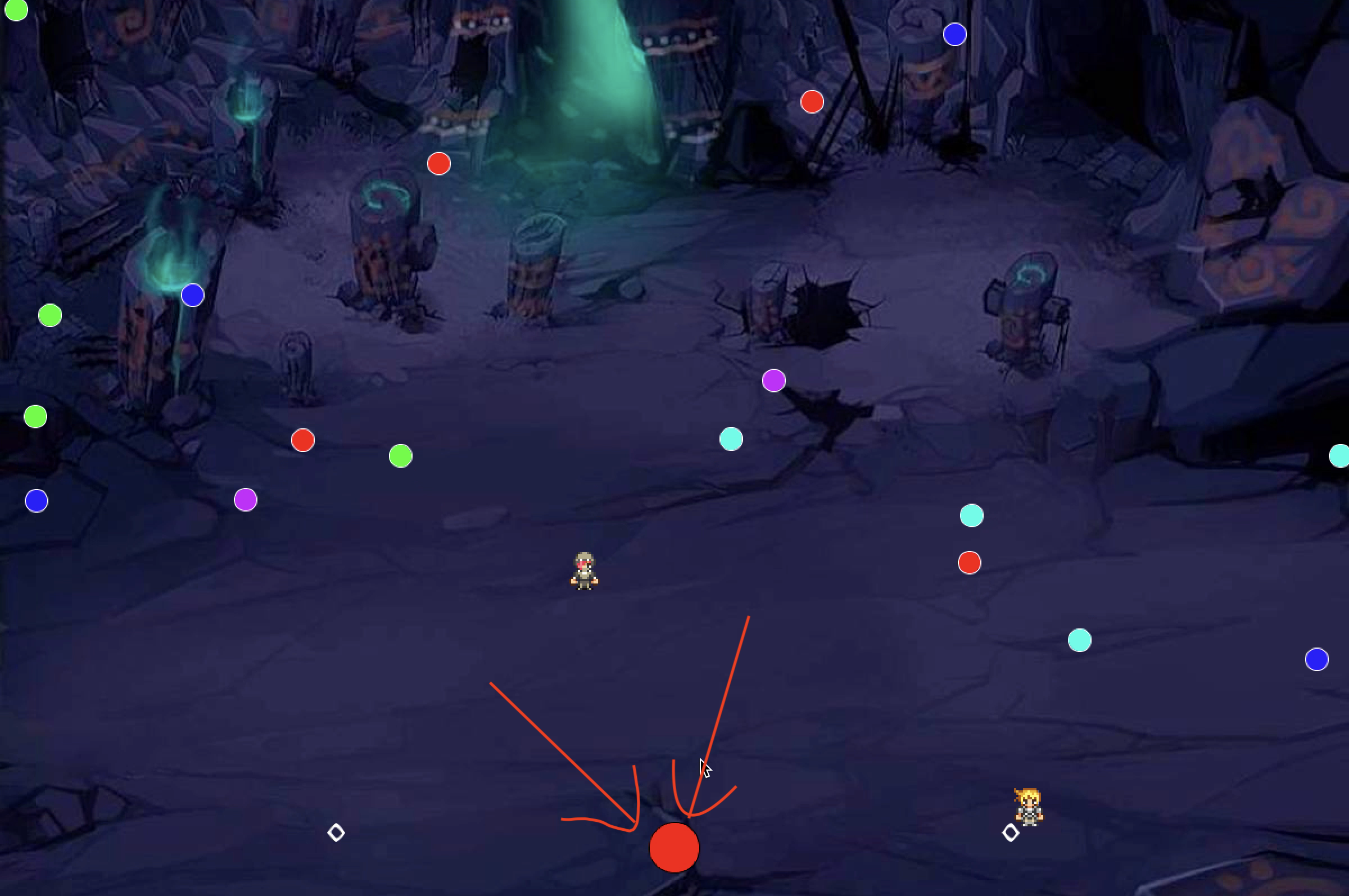If a player “eats” an edible candy, one point will go to their score, which shows at their spawn point, at the bottom of the screen. If a player “eats” a poisonous candy, they die and respawn at their spawn point. Once all the edible candies are collected, the player with the most candy wins. If both have the same amount, it is a tie.

When the game initializes, there is a starting screen with the name of the game, “Candy Rush”, as well as the instructions and a “play” button. When hovering the mouse over the play button, the button will be highlighted: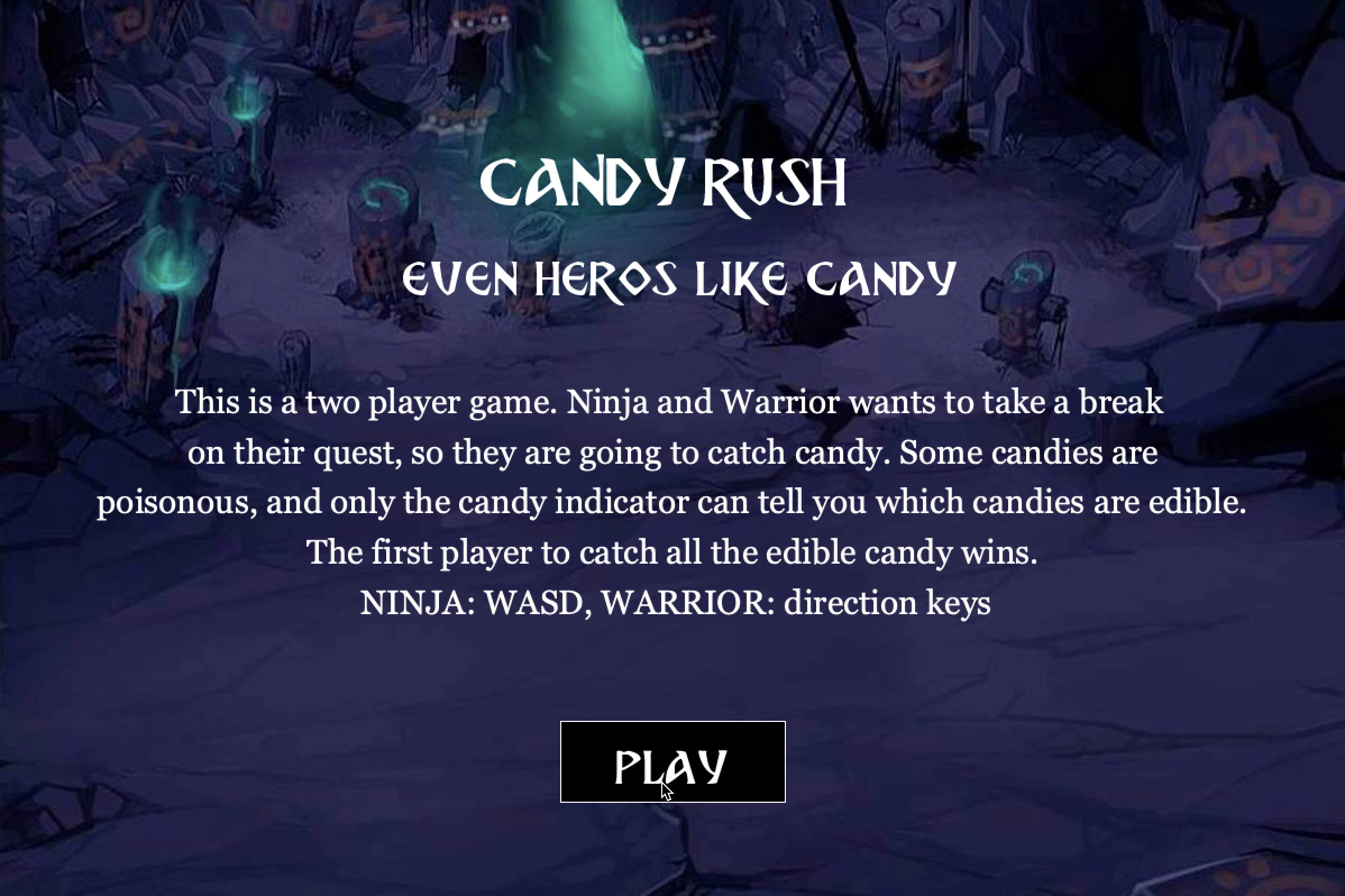Regarding the sounds, the game has three in total. One is the background music that loops endlessly. The other two are sounds for when the hero eats a poisonous candy and an edible candy.

There are two classes, one for the heroes and one for the candies. The hero tab simply includes its display and move functions. The candy tab includes its display, collision with the heroes, collision with a wall, and movement functions. The main code page, apart from draw and setup, includes four main functions. The reset function is in charge of initializing all the game values. Most of these functions could have gone into setup, but I needed to create a restart function for when the game ends. Thus, I moved most of the value initialization into the reset function, and then called the reset function in setup. This way, I could decide when to “re-setup” my game.

The next function is menu, which is in charge of the starting menu. I run this function first in draw. While running the menu function, when the player clicks “play”, the universal boolean “go” will turn to true, triggering the begin function.

The begin function is the main gameplay, where all the object functions are called. At the end of the begin function, the last function is called, which is checkwin.

Checkwin simply runs through the win conditions constantly and displays the winning screen when the win condition is met.

Images used

Background: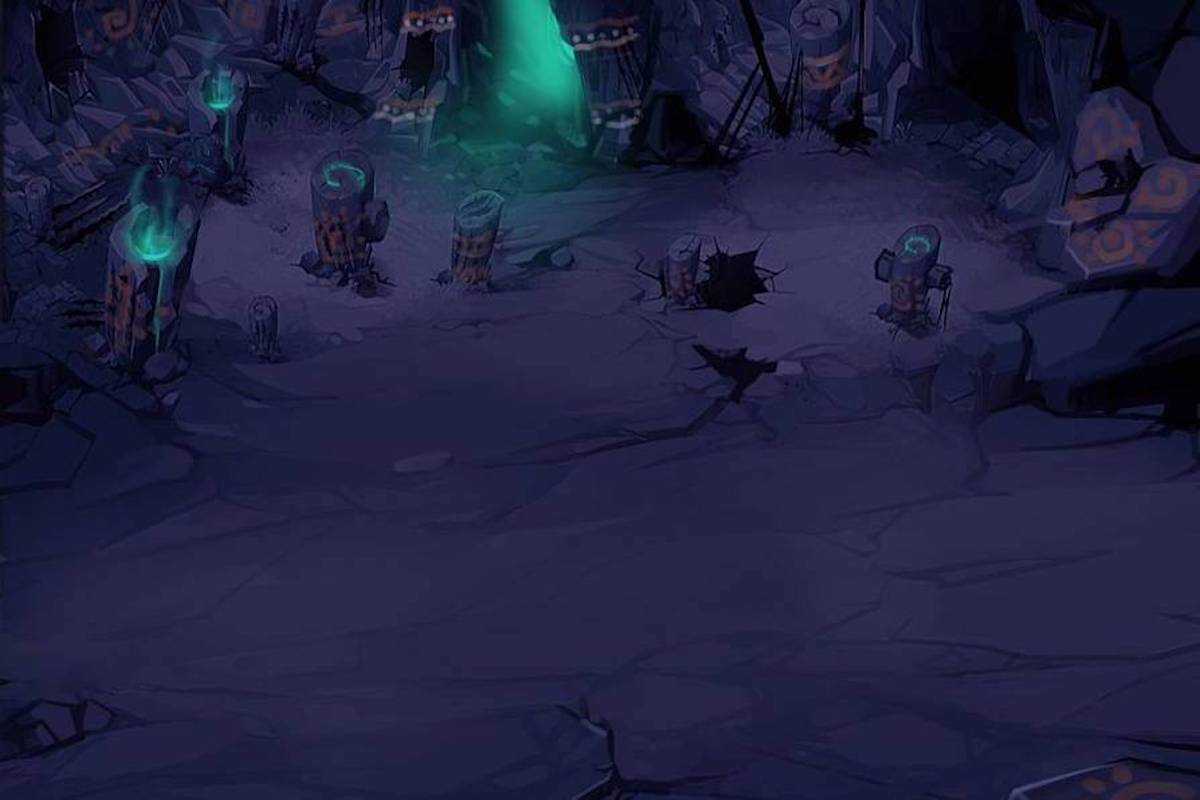Hero Sprites: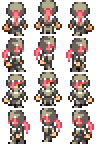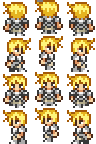Sounds used

Background Music:

Catching edible candy:

Catching poisonous candy:

Full Code

Main Code Page:

```PFont font;

import processing.sound.*;            //all the sounds in this game
SoundFile eat;
SoundFile die;
SoundFile music;

Ball b1;                              //initializing the two players
Ball b2;
PImage ninja, warrior, bg;
PImage[][] ninjawalk;
PImage[][] warriorwalk;
int ndirection = 0;
int wdirection = 0;
int nstep = 0;
int wstep = 0;
int speed = 5;

Candy[] candy;                        //initializing all the candy
int count;
color chosen;
int livecount = 0;                    //how many edible candy there are

boolean go = false;                   //start game value

void setup() {
size(1200, 800);

//sounds
eat = new SoundFile(this, "eat.mp3");
die = new SoundFile(this, "die.mp3");
music = new SoundFile(this, "music.mp3");
music.loop();

//slicing the two sprite sheets for player 1 and 2
ninjawalk = new PImage;
int w = ninja.width/3;
int h = ninja.height/4;
for(int iy = 0; iy < 4; iy++){
for(int ix = 0; ix<3; ix++){
ninjawalk[iy][ix] = ninja.get(ix*w, iy *h, w, h);
}
}
warriorwalk = new PImage;
int ww = warrior.width/3;
int wh = warrior.height/4;
for(int wy = 0; wy < 4; wy++){
for(int wx = 0; wx<3; wx++){
warriorwalk[wy][wx] = warrior.get(wx*ww, wy *wh, ww, wh);
}
}
//use this function to restart the game
reset();
}

void draw() {
if (go == true){
begin();
}
}

//this function restarts the game from the gaming interface directly
void reset(){
float unit = 200;
b1 = new Ball(width/4, height - 20, 1);          //player 1 character
b2 = new Ball(width*3/4, height - 20, 2);        //player 2 character

int wideCount = width / int(unit);               //calling the candies, initializing different candy colors
int highCount = (height*3/4) / int(unit);
count = wideCount * highCount;
candy = new Candy[count];
int index = 0;
color[] colors = new color;
colors = #FF0011;
colors = #BA00FF;
colors = #2500FF;
colors = #00FFE8;
colors = #00FF01;
colors = #FFAF00;
chosen = colors[int(random(0,5))];
for (int y = 0; y < highCount; y++) {
for (int x = 0; x < wideCount; x++) {
candy[index++] = new Candy(x * unit + unit/2, y * unit + unit/2, 20, 20, colors[int(random(0,5))]);
}
}

for (Candy candy : candy) {                      //counting how many edible candies there are to know the win condition
if (chosen == candy.c){
livecount ++;
}
}

for (int i =0; i<count;i++){                     //candy speed initializing
candy[i].xSpeed = random(-2,2);
candy[i].ySpeed = random(-2,2);
}
}

background(bg);
fill(255);
font = createFont("BreatheFire-65pg.ttf", 60);
textFont(font);
textAlign(CENTER);
text("CANDY RUSH \n even heros like candy", width/2, 185);
font = createFont("Georgia", 40);
textFont(font, 30);
text("This is a two player game. Ninja and Warrior wants to take a break \n on their quest, so they are going to catch candy. Some candies are \n poisonous, and only the candy indicator can tell you which candies are edible. \n The first player to catch all the edible candy wins. \n NINJA: WASD, WARRIOR: direction keys", width /2, 370);
stroke(0);
fill(0);
rectMode(CENTER);
rect(width/2, 680, 200, 72);
fill(255);
textFont(font, 60);
font = createFont("BreatheFire-65pg.ttf", 42);
textFont(font);
textAlign(CENTER);
text("PLAY", width/2, 700);
if (mouseX>=(width/2 - 100)  & mouseX<=(width/2 + 100) & mouseY>=650 & mouseY<=720){
stroke(255);
noFill();
rect(width/2, 680, 200, 72);
if (mousePressed){
go = true;
}
}
}

//this is the game starting function. the actual game is ran here
void begin(){
background(bg);
textSize(24);
text(b1.score, width/4, height - 50);
text(b2.score, width*3/4, height - 50);

//calling candy functions
for (Candy candy : candy) {
candy.display();
candy.collision();
candy.update();
candy.checkEdges();
}

//this is the candy indicator
stroke(0);
fill(chosen);
ellipse(width/2, height - b1.diameter, b1.diameter, b1.diameter);

//calling character functions
b1.display();
b1.move();
b2.display();
b2.move();
checkwin();
}

void checkwin(){
if (livecount == 0){                      //win condition
for (Candy candy : candy) {
candy.xSpeed = 0;
candy.ySpeed = 0;
}
rectMode(CENTER);
if (b1.score > b2.score){
fill(0);
rect(width/2, height/2, width, height);
fill(255, 255, 255);
textSize(32);
text("Ninja Wins! \n (Press r to restart)", width/2, height/2);
}
else if (b2.score > b1.score){
fill(0);
rect(width/2, height/2, width, height);
fill(255, 255, 255);
textSize(32);
text("Warrior Wins! \n (Press r to restart)", width/2, height/2);
}
else if (b2.score == b1.score){
fill(0);
rect(width/2, height/2, width, height);
fill(255, 255, 255);
textSize(32);
text("Tie! \n (Press r to restart)", width/2, height/2);
}

//restart button
if (keyPressed) {
if (key == 'r' || key == 'R') {
reset();
}
}
}
}```

Player Class Page:

```class Ball {

float x;
float y;
float diameter;
int score;
int player;
int w = ninja.width/3;
int h = ninja.height/4;
int ww = warrior.width/3;
int wh = warrior.height/4;

Ball(float tempX, float tempY, int tempPlayer) {
x = tempX;
y = tempY;
diameter = 45;
score = 0;
player = tempPlayer;
}

void display() {
imageMode(CENTER);                                    //displaying player 1
if (player == 1){
image(ninjawalk[ndirection][nstep],x,y,w,h);
}
if (player == 2){                                     //displaying player 2
image(warriorwalk[wdirection][wstep],x,y,ww,wh);
}
}

void move() {
if (player == 1){
if (keyPressed) {
if (key == 'w' || key == 'W') {
y = y - 5;
ndirection = 0;
}
if (key == 'a' || key == 'A') {
x = x - 5;
ndirection = 3;
}
if (key == 's' || key == 'S') {
y = y + 5;
ndirection = 2;
}
if (key == 'd' || key == 'D') {
x = x + 5;
ndirection = 1;
}
if (frameCount%speed == 0) {
nstep = (nstep + 1) %3;
}
}
}

if (player == 2){
if (keyPressed) {
if (keyCode == UP) {
y = y - 5;
wdirection = 0;
}
if (keyCode == LEFT) {
x = x - 5;
wdirection = 3;
}
if (keyCode == DOWN) {
y = y + 5;
wdirection = 2;
}
if (keyCode == RIGHT) {
x = x + 5;
wdirection = 1;
}
if (frameCount%speed == 0) {
wstep = (wstep + 1) %3;
}
}
}
}
}```

Candy Class Page:

```class Candy {
float x;
float y;
float w;
float h;
float xSpeed, ySpeed;
boolean caught = false;
color c;

Candy(float tempX, float tempY, float tempW, float tempH, color tempC) {
x = tempX;
y = tempY;
w = tempW;
h = tempH;
c = tempC;
xSpeed = ySpeed = 0;
}

void display() {

ellipseMode(CENTER);
if (caught == false){
fill(c);
ellipse(x, y, w, h);
}
}

//collision function for when players catch the right and wrong candy
void collision() {
if ((b1.x - b1.w *3/4 < x && x < b1.x + b1.w*3/4) & (b1.y - b1.h*3/4 < y && y < b1.y + b1.h*3/4)){
if (c == chosen){
x = -50;
y = -50;
caught = true;
b1.score ++;
livecount --;
eat.play();
}
else if (c != chosen){
b1.x = width/4;
b1.y = height - b1.diameter;
die.play();
}
}
if ((b2.x - b2.w*3/4 < x && x < b2.x + b2.w*3/4) & (b2.y - b2.h*3/4 < y && y < b2.y + b2.h*3/4)){
if (c == chosen){
x = -50;
y = -50;
caught = true;
b2.score ++;
livecount --;
eat.play();
}
else if (c != chosen){
b2.x = width*3/4;
b2.y = height - 20;
die.play();
}
}
}

void update(){
x += xSpeed;
y += ySpeed;
}

void checkEdges() {
if (y>(height*3)/4) {
ySpeed = -ySpeed;
}
if (y<0) {
ySpeed = -ySpeed;
}
if (x>width) {
xSpeed = -xSpeed;
}
if (x<0) {
xSpeed = -xSpeed;
}
}
}```

Entire Compressed Code

Midterm

Game Demonstration

## Image Manipulation – Lakers 2020

I’ve been a Kobe fan for forever and a Lakers fan since 2012, and it’s been a hard decade for the team. hard. So, in light of the Lakers winning the 2020 NBA championship today, I wanted to do something with image manipulation that was related to the lakers.

My idea is to fill the screen with the laker logo, and every time I draw on the screen via my mouse, another image would appear with tints and effects. This way I can draw stuff like “2020 champs” etc etc.

I do feel kind of weird about the project, I felt like to do what I was doing, there were more object-oriented programming than image manipulation. Perhaps next time it might be better to focus on one image and changing its properties than to change a whole bunch of images.

```PImage img, lebron;
float unit = 30;    //unit of each picture
int count;          //how many pictures are there in a certain sized frame
Ring[] ring;

void setup(){
size(1500, 900);
int wideCount = width / int(unit);
int highCount = height / int(unit);
count = wideCount * highCount;

//calling an array of image objects with the width and height counts
ring = new Ring[count];
int index = 0;
for (int y = 0; y < highCount; y++) {
for (int x = 0; x < wideCount; x++) {
ring[index++] = new Ring(x * unit, y * unit, width/unit, width/unit);
}
}

}

void draw(){
//running the functions for every image
for (Ring ring : ring) {
ring.display();
ring.update();

}

}```
```class Ring {
float x;
float y;
float w;
float h;

//for images that have been hovered over
boolean changed = false;

Ring(float tempX, float tempY, float tempW, float tempH) {
x = tempX;
y = tempY;
w = tempW;
h = tempH;
}

void display() {
//original image
if (changed == false){
noTint();
image(img, x , y, w, h);
}
//changed images
if (changed == true){
tint(165, 111, 124, 50);
image(lebron, x, y, w, h);
}
}

void update() {
//checking if an image is clicked on
if (mousePressed){
if (x < mouseX && mouseX < x+unit && mouseY > y && mouseY < y + unit){
changed = true;
}
}
}
}```

## Generative text assignment

In last week’s class, I was really interested in the circle of letters code, so I wanted to do something similar for my generative text assignment. I borrowed a lot of code from the circle code document, and in this week’s assignment, a lot of my time was put into studying the code in order to apply the additions that I wanted to.

Regarding my idea, the circle of letters reminded me of Iron man 1, when Pepper gave Tony the gift that said: “Proof that Tony Stark has a heart.” Our assignment was to create generative texts, so I couldn’t just create a stagnant version of the gift. Instead, I decided to make it blink, like how tony’s reactor would blink sometimes.

Code wise, some lines in the code was really helpful in helping me understand arrays and objects. For instance:

`Circle letters[] = new Circle[s.length()];`

I was still unfamiliar with calling multiple objects and putting them into arrays, so this line helped clear things up for me well. The use of trigonometry was also inspiring for my future approaches to OOP.

Here is my full code:

```String s = "        PROOF THAT TONY STARK                    TRAEH A SAH            ";
PImage img;
float speed = 2;

Circle letters[] = new Circle[s.length()];
PFont f;
float r = 250;
float dir = 1;
float font_size = 30;

void setup(){
size(640, 640);
}

void draw(){
background(0);
f = createFont("Arial", font_size);
textFont(f);
float startingAngle = PI;
float circleCenterX = width/2;
float circleCenterY = height/2;

for (int i =0; i<s.length();i++){
float angle = startingAngle + i*TWO_PI/s.length();
float x = cos(angle)*radius + circleCenterX;
float y = sin(angle)*radius + circleCenterY;
letters[i] = new Circle(x, y, s.charAt(i));
}

for (int i =0; i<s.length();i++){
letters[i].display();
}

if (r >=  250){
dir = -dir;
}
else if (r <= 20){
dir = 1;
}
imageMode(CENTER);
image(img, width/2+font_size/3, height/2-font_size/3,  r*2-font_size, r*2-font_size);

if (dir == -1){
font_size = font_size - speed/8;
}
else if (dir == 1){
font_size = font_size + speed/8;
}

r = r + speed * dir;

}```

Circle class:

```class Circle {
float x, y;
char letter;

Circle(float _x, float _y, char _c) {
x = _x;
y = _y;
letter = _c;
}

void display() {
fill(255);
text(letter,x,y);
}
}```

Video: